Bagikan Artikel ini di:

Despite having a very significant influence in bridging the classical era into the modern era and is called one of the most influential physicists ever to exist with Isaac Newton and Albert Einstein, few people are familiar with James Clerk Maxwell commonly known as Maxwell. His greatness made Maxwell the foundation for Mr. Relativity, Albert Einstein, in developing his modern ideas expressed in the following sentence: ” I stand not on the shoulders of Newton, but on the shoulders of James Clerk Maxwell “.

Born on June 13, 1831 in Edinburgh, Scotland, since childhood Clerk Maxwell already has a great sense of curiosity about how something can happen and work. This curiosity led Maxwell’s 14-year-old to complete his first paper on oval geometry entitled On the Description of Oval Curves, and Those Having a Plurality of Foci read by the Royal Society of Edinburgh on April 6, 1846. Maxwell had a college education at University of Edinburghthen proceeded at Cambridge University’s Trinity College which he completed in 1854 and was selected as Chair of Natural Philosophy atUniversity of Aberden at the age of 25 years. He is known for his research on the field of kinetic theory and thermodynamics, optics, and his greatest breakthrough in the field of electromagnetism projected into the Maxwell Equations.

Maxwell has a remarkable ability in performing mathematical abstractions to explain a physical condition. This can be seen in his analysis of the stability of the saturnus rings he describes using mathematics by predicting that the Saturn rings are composed of a set of tiny particles in very large quantities, if the farther from the center of mass saturn, the less the number of particles is due to the influence of increasing Saturnian gravity small. Then after more than a hundred years later that is in 2004, the new theory was successfully proven by NASA.

Maxwell’s ability to describe statistical behavior on classical gas molecules and his most beautiful works in explaining the symmetry of electricity and magnetism as electromagnetic waves and mathematical explanations that tell that light is an electromagnetic wave; shows Maxwell’s unquestionable genius as a physicist whose influence stands side by side with Newton and Einstein.

If talking about the technology around us then can not be separated from the great contribution of the Maestro Electromagnetism. From the start of radio, television, radar, wifi and bluetooth are some examples of current technology that beranasakan theory of electromagnetic field that has been summarized by Clerk Maxwell into four Maxwell equations. Physics World magazine , one of the largest physics magazines in the world that covers all areas of physics, chose Maxwell’s Equation as ” the most important equations of all time “.

From the beginning is often called the term ‘electromagnetism’, what exactly is electromagnetism? Electromagnetism is a process that explains the linkage between the electric field and the magnetic field that includes its propagation, its interaction, and how the two fields affect other objects. The major principles in electromagnetism have been summarized by Maxwell into his four famous equations which will try to explain the author simply without complex mathematics.

1. Gauss’s Law

Maxwell’s first equation is Gauss’s law. Gauss’ law explains the behavior of the electric field around the electrical charge.The above equation is a manifestation of Gauss’ law. The right-hand side of the equation shows the type of volume charge ρ v that describes how tightly the set of charges in a given volume; then the left side of the equation showing a radiant electric field D that is symmetrical in all directions.Figure 2. Emission of electric field lines in the plane (a) positive charge, (b) negative charge (https://courses.lumenlearning.com/physics/chapter/18-5-electric-field-lines-multiple-charges /)

It can be concluded that there will be a symmetrical (symmetrically visualized) electric field radiated in any direction through a volume field sourced from a certain amount of electrical charge in the volume field (the emission will come out if the charge is positive and will go in if the charge electricity negative). Or simply, if there is an electrical charge in a certain position there will be electric field radiation in all directions. A plurality of electric field jets (illustrated by field lines) penetrating the volume field will be denser if the charge is multiplied (provided that the load in the volume has the same pole that is positive or negative only if there are several charges with different poles then the field of electric field will reduce each other) or the volume is reduced which meansits value will be greater. This is the theory and the mathematical form that underlies the explanation of the electric field direction of the positive and negative charges that have been studied since junior high.

1. Law of Gauss Magnetism

The Law of Magnetism Gauss states that the total jets of magnetic field lines penetrating a given volume are zero ( B is a magnetic field).This happens because the magnet must have two poles (magnetic dipole) or no magnet that has only one pole (no magnetic monopol). Then why in the absence of a magnetic monopolist causes the total emission of a magnetic field penetrating a given volume is zero? Yep the following explanation.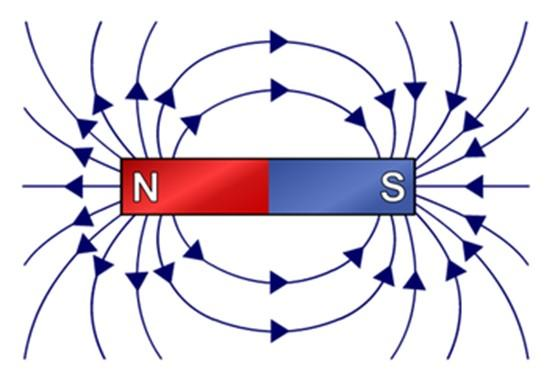Figure 3. Emission of magnetic field lines in a magnetic dipole (https://brilliant.org/wiki/magnetic-field-lines/)

The absence of a monopole magnetic means the presence of a magnetic dipole. Magnetic dipole shows that the magnet will always have two poles of the north and south poles; and it is impossible to consist of only the north pole or the south pole only. We know that the direction of the magnetic field lines from the north pole to the south pole. Imagine we put a magnet inside a cube. After that, the magnetic field lines will come out of the north pole, pierce one side of the cube (call it A side) and then enter through the opposite side of the cube (call it B side) to the south pole. The number of magnetic field lines radiating out from side A will be equal to the number of magnetic field lines radiating in from side B; so the total emission of the magnetic field lines will be equal to zero.

Now we go into the third Maxwell Equation that is Faraday’s law. Faraday was an experimental physicist who succeeded in proving that the speed of changes in the magnetic field and the effective cross-sectional area (the effective cross-sectional area is the area penetrated perpendicularly by the magnetic field) would produce induced electrical current and induced voltage (induced electrical current and induced voltage are terms for the current and the electric voltage generated through the change of the magnetic field per unit of time, or in this case, the word induction can also be understood along with the word stimulation). The magnitude of the magnetic flux that is the scalar multiplication of both magnitudes. Then Lenz complements Faraday’s law by saying thatpolarity (induced poles) of induced voltage is such that the induced voltage tends to produce currents that give rise to a magnetic field (the lenz field) against the change of magnetic flux through the loop ; the statement is referred to as Lenz’s law. So the mathematical form of Faraday’s law is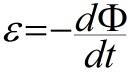with ε and Φ are the induced voltages and magnetic flux respectively. The negative sign (the result of Lenz’s law), indicates that the resulting opposite poles of the induced voltage will produce an electric current in the opposite direction causing the magnetic field lines to be produced in the opposite direction of the initial magnetic flux to act as the inducer. The opposite here means that imagine a magnetic field coming from the smartphone screenor our laptop with the magnitude that changes with the lines of the field towards us. Then by clenching the four fingers of our right hand (as the direction of the electric current) and the thumb pointing towards us (as the direction of the magnetic field lines) we will be able to know the direction of the resulting electric current that is (supposedly anticlockwise). However, the direction of the resulting induced electrical current is opposite from that, ie clockwise with the positive and negative poles of the induced voltage adjusting because the electrical current visualization is flowing from the positive pole to the negative pole. The direction of the current and induced magnetic field can be known by the left hand rule (as opposed to as it should be, please try!).

By associating the equation with the relationship between the electric-electric field voltage; and the Stokes theorem is obtained the following equation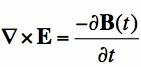Baca juga:

The above equation is the third Maxwell equation. Whew, starting from the connection of electrical voltage, electric current, and magnetic field; it turns out the relationship between electric field E and magnetic field B ! (Here is not explained how the mathematical process, because it is long enough).

The interpretation of the third Maxwell equation above is that the magnetic field changes will produce an electric field whose direction of electric field lines surrounds the magnetic field lines (perpendicular to each other) with the relationship between the direction of the two fields can be determined by the left hand rule (four fingers the left hand is clenched and the thumb is fixed The direction of the thumb shows the direction of the magnetic field and the fist of the other four fingers indicates the direction of the electric field surrounding the magnetic field).

1. Ampere-Maxwell’s Law

Finally, we have reached the last Maxwell equation which will explain that it turns out that not only changes in the magnetic field can produce an electric field, but changes in electric fields can also produce changes in magnetic fields! Although the basis of Maxwell’s fourth equation is the law of Ampere but Maxwell is the one who sparked the idea of ​​the symmetry between the electric field and the magnetic field (Briefly symmetry can be understood as the action of reaction If X then Y, then vice versa if Y then X). The idea of ​​Maxwell is simple, he knows that in this world symmetry plays an important role. So if a magnetic field change can produce an electric field then a change of electric field will certainly produce a magnetic field.

Based on his experimental results, Ampere found that when there is an electric current flowing in a conductor it will produce a magnetic field that surrounds the electric current is based on the rules of the right hand (the thumb as the direction of electric current and the fourth fist finger other as the direction of the magnetic field); and based on a sharp intuition equipped with mathematics, Maxwell sparked that changes in the electric field would produce a magnetic field. The idea is intertwined with another idea which states that the electric current can not only flow on the conductor but also can flow on the insulator called the displacement current . The shifting current is generated from changes in the electric field.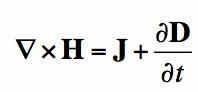The above equation is the fourth Maxwell equation by J is the electric current density is the amount of electric current that flows per unit area, and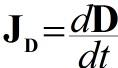the flow shifts resulting from changes in the electric field D . Interpretation of the fourth Maxwell equation as follows, the electric current flowing in the conductor J will produce an H magnetic field that travels around the conductor and if the electric field D within the conductor is altered it will produce a D shift current which also has a contribution in generating magnetic field around it.So what if in the isolator medium like a vacuum or air? Then the electric current J will be lost so as to produce the form of the fourth Maxwell equation in a vacuum that is only influenced by changes in electric field (current shift),Perhaps this pure contribution from Maxwell looks small, but in fact this contribution is enormous. The idea of ​​the symmetry of the electric field and the magnetic field is what unites the concept of all equations in electricity and magnet so that the term ‘electromagnetism’ occurs; then this idea is also the basis of the concept in explaining the propagation of electromagnetic waves until finally found that the propagation speed of electromagnetic waves always the same if it is on the same medium that is the speed of light propagation. So it can be concluded that light is a new electromagnetic wave can be proven through experiments by Heinrich Hertz in 1888, 9 years after the death of Maxwell.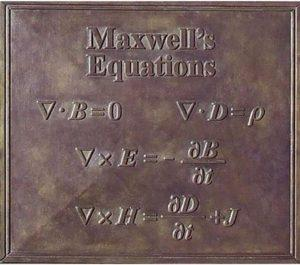Figure 4. The monument of four Maxwell equations in the theory of electromagnetism (https://en.wikipedia.org/wiki/James_Clerk_Maxwell_Foundation)

In addition, the development timeline follows from the theory of electromagnetism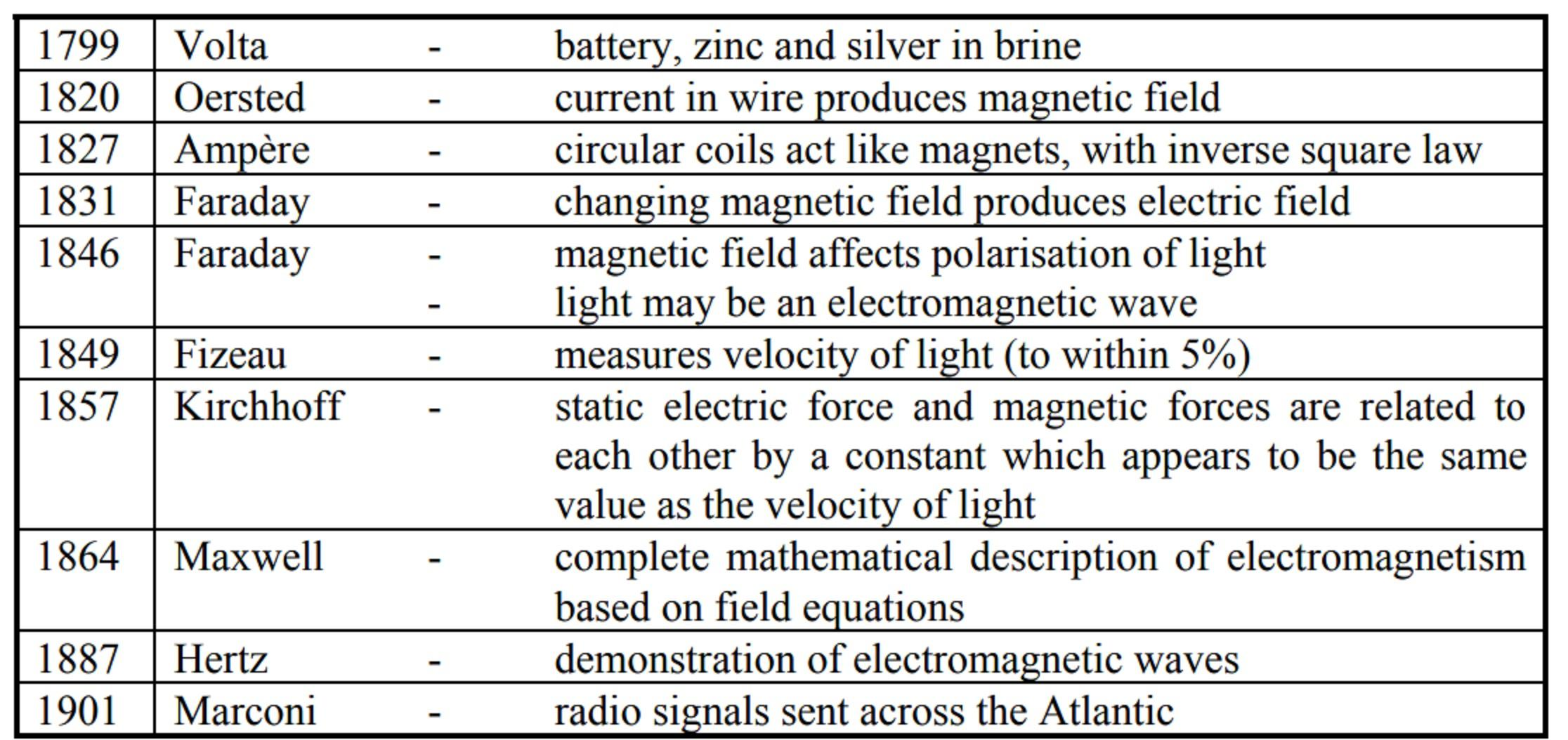Figure 5. Ten physicists who contributed to the development of electromagnetism theory from the late 18th century to early 20th century (http://www.clerkmaxwellfoundation.org/Maker_of_Waves.pdf)

Of the 10 physicists who contribute to Maxwell’s development of electromagnetism theory only contributes to the mathematical descriptions (theoretically) as well as the only ones who can provide precise predictions about new concepts and ideas before experiments are successful.

For the related explanations of how the Maxwell concept and equations can prove that light is an electromagnetic wave will be discussed in an upcoming article. See ya soon !

#### Reference

1. A & E Television Networks. (2015, 24 June). James C. Maxwell Biography. Retrieved 6 April 2018, from https://www.biography.com/people/james-c-maxwell-9403463
2. James Clerk Maxwell Foundation. “__”. Maker of Waves. Retrieved 6 April 2018, from http://www.clerkmaxwellfoundation.org/Maker_of_Waves.pdf
3. James Clerk Maxwell Foundation. “__”. Saturn’s Rings. Retrieved 6 April 2018, from http://www.clerkmaxwellfoundation.org/Saturn-s_Rings.pdf
4. JJ O’Connor and EF Robertson. “__”. James Clerk Maxwell. Retrieved 6 April 2018, from http://www-groups.dcs.st-and.ac.uk/history/Biographies/Maxwell.html
5. Maxwells-Equations. (2012). Ampere’s Law. Retrieved 6 April 2018, from http://www.maxwells-equations.com/ampere/amperes-law.php
7. Maxwells-Equations. (2012). Gauss’ Law. Retrieved 6 April 2018, from http://www.maxwells-equations.com/gauss/law.php.
8. Maxwells-Equations. (2012). Gauss’ Law for Magnetism. Retrieved 6 April 2018, from http://www.maxwells-equations.com/gauss/magnetism.php
9. Sci-Show. (2016, 15 September). Great Minds: James Clerk Maxwell, Electromagnetic Hero. Retrieved 5 April 2018, from https://www.youtube.com/watch?v=b2cVLHozb9k
10. Surya, Yohannes. 2009. Series of Materials for Physics Olympiad Preparation: Electricity and Magnetism. Tangerang: Kandel.
11. Tiger Webb. (2015, 1 December). James Clerk Maxwell: The Greatest Physicist You’ve Never Heard of. Retrieved 6 April 2018, from http://www.abc.net.au/radionational/programs/scienceshow/james-clerk-maxwell:-the-greatest-physicist/6990508
12. Wikipedia. (2018, 30 March). James Clerk Maxwell. Retrieved 6 April 2018, from https://en.wikipedia.org/wiki/James_Clerk_Maxwell

### Warstek Media

Warung Sains Teknologi (Warstek) adalah media SAINS POPULER yang dibuat untuk seluruh masyarakat Indonesia baik kalangan akademisi, masyarakat sipil, atau industri. Sampai saat ini, sains dan teknologi berkesan ekslusif yang hanya ada di laboratorium dan tidak mampu secara langsung berdampak kepada masyarakat. Akibatnya masyarakat, pemerintah dan industri tidak menjadikan sains sebagai sarana mengatasi permasalahan atau membuat kebijakan. Untuk itu, warstek hadir untuk menjawab tantangan tersebut.
 Nilai Artikel Ini
Artikel Berhubungan: